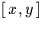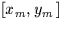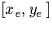Next: SLA_FK425 - FK4 to FK5
Up: SUBPROGRAM SPECIFICATIONS
Previous: SLA_EVP - Earth Position & Velocity

## SLA_FITXY - Fit Linear Model to TwoSets

ACTION:
Fit a linear model to relate two sets ofcoordinates.
CALL:
CALL sla_FITXY (ITYPE,NP,XYE,XYM,COEFFS,J)

GIVEN:

 I ITYPE type of model: 4 or 6 (note 1) NP I number of samples (note 2) XYE D(2,NP) expectedfor each sample XYM D(2,NP) measuredfor each sample

RETURNED:

 D(6) COEFFS coefficients of model (note 3) J I status: 0 = OK -1 = illegal ITYPE -2 = insufficient data -3 = singular solution

NOTES:
1.
ITYPE, which must be either 4 or 6, selects the type of model fitted. Both allowed ITYPE values produce a model COEFFS which consists of six coefficients, namely the zero points and, for each of XE and YE, the coefficient of XM and YM. For ITYPE=6, all six coefficients are independent, modelling squash and shear as well as origin, scale, and orientation. However, ITYPE=4 selects the solid body rotation option; the model COEFFS still consists of the same six coefficients, but now two of them are used twice (appropriately signed). Origin, scale and orientation are still modelled, but not squash or shear - the units of X and Y have to be the same.
2.
For NC=4, NP must be at least 2. For NC=6, NP must be at least 3.
3.
The model is returned in the array COEFFS. Naming the six elements of COEFFS a,b,c,d,e & f, the model transforms measured coordinatesinto expected coordinatesas follows:
xe = a + bxm + cym
ye = d + exm + fym
For the solid body rotation option (ITYPE=4), the magnitudes of b and f, and of c and e, are equal. The signs of these coefficients depend on whether there is a sign reversal between [xe,ye] and [xm,ym]; fits are performed with and without a sign reversal and the best one chosen.
4.
Error status values J=-1 and -2 leave COEFFS unchanged; if J=-3 COEFFS may have been changed.
5.